Courses

# Test: CAT Quant 2018 (Shift - 1)

## 34 Questions MCQ Test Quantitative Aptitude (Quant) | Test: CAT Quant 2018 (Shift - 1)

Description
This mock test of Test: CAT Quant 2018 (Shift - 1) for CAT helps you for every CAT entrance exam. This contains 34 Multiple Choice Questions for CAT Test: CAT Quant 2018 (Shift - 1) (mcq) to study with solutions a complete question bank. The solved questions answers in this Test: CAT Quant 2018 (Shift - 1) quiz give you a good mix of easy questions and tough questions. CAT students definitely take this Test: CAT Quant 2018 (Shift - 1) exercise for a better result in the exam. You can find other Test: CAT Quant 2018 (Shift - 1) extra questions, long questions & short questions for CAT on EduRev as well by searching above.
QUESTION: 1

### If x is a positive quantity such that 2x = 3log52.then x is equal to

Solution: Given that: 2x = 3log5 2

=> 2x = 2log53

=>x = log53

=> x = log5 (3 * 5 / 5)

=> x = log5 5 + log5 (3 / 5)

=> x = 1 + log5 5

Hence, option D is the correct answer.

QUESTION: 2

### In a circle, two parallel chords on the same side of a diameter have lengths 4 cm and 6 cm. If the distance between these chords is 1 cm, then the radius of the circle, in cm, is

Solution: Given that two parallel chords on the same side of a diameter have lengths 4 cm and 6 cm.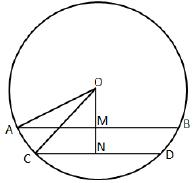In the diagram we can see that AB = 6 cm, CD = 4 cm and MN = 1 cm.

We can see that M and N are the mid points of AB and CD respectively.

AM = 3 cm and CD = 2 cm. Let 'OM' be x cm.

In right angle triangle AMO,

AO2 = AM2 + OM2

=> AO2 = 32 + x2 …. (1)

In right angle triangle CNO,

CO2 = CN2 + ON2

=> CO2 = 22 + (OM + MN)2

=> CO2 = 22 + (x + 1)2 …..(2)

We know that both AO and CO are the radius of the circle. Hence AO = CO

Therefore, we can equate equation (1) and (2)

32 + x2 = 22 + (x+1)2

= x = 2cm

Therefore, the radius of the circle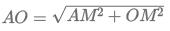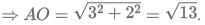Hence, option A is the correct answer.

QUESTION: 3

### Humans and robots can both perform a job but at different efficiencies. Fifteen humans and five robots working together take thirty days to finish the job, whereas five humans and fifteen robots working together take sixty days to finish it.Q. How many days will fifteen humans working together (without any robot) take to finish it?

Solution: Let the efficiency of humans be 'h' and the efficiency of robots be 'r'.

In the first case,

Total work = (15h + 5r) * 30......(i)

In the second case,

Total work = (5h + 15r) * 60......(ii)

On equating (i) and (ii), we get

(15h + 5r) * 30 = (5h + 15r) * 60

Or, 15h + 5r = 10h + 30r

Or, 5h = 25r

Or, h = 5r

Total work = (15h + 5r) * 30 = (15h + h) * 30 = 480h

Time taken by 15 humans = days= 32 days.

Hence, option C is the correct answer.

QUESTION: 4

Let ABCD be a rectangle inscribed in a circle of radius 13 cm. Which one of the following pairs can represent, in cm, the possible length and breadth of ABCD?

Solution: Let ABCD be a rectangle inscribed in a circle of radius 13 cm. Which one of the following pairs can represent, in cm, the possible length and breadth of ABCD?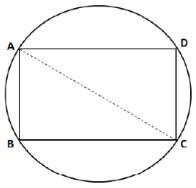We know that AC is the diameter and

In right angle triangle ABC,

AC2 = AB2 + BC2

⇒ AB2 + BC2 = 262

⇒ AB2 + BC2 = 676

Let us check with the options.

Option (A): 242 + 102 = 676. Hence, this is a possible answer.

Option (B): 252 + 92 = 706 ≠ 676. Hence, this is an incorrect pair.

Option (C): 252 + 102 = 725 ≠ 676. Hence, this is an incorrect pair.

Option (D): 252 + 122 = 720 ≠ 676. Hence, this is an incorrect pair.

Therefore, we can say that option A is the correct answer.

QUESTION: 5

Points E, F, G, H lie on the sides AB, BC, CD, and DA, respectively, of a square ABCD. If EFGH is also a square whose area is 62.5% of that of ABCD and CG is longer than EB, then the ratio of length of EB to that of CG is

Solution: It is given that EFGH is also a square whose area is 62.5% of that of ABCD. Let us assume that E divides AB in x : 1. Because of symmetry we can say that points F, G and H divide BC, CD and DA in x : 1. Let us assume that 'x+1' is the length of side of square ABCD.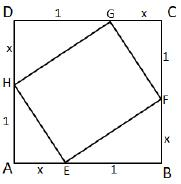Area of square ABCD = (x+1)2 sq.units

Therefore, area of square EFGH = 62.5 / 100 * (x + 1)2 = 5(x + 1)2 / 8

In right angle triangle EBF,

EF2 = EB2 + BF2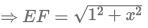Therefore, the area of square EFGH = EF2 = x2 + 1 ... (2)

By equating (1) and (2),

x2 + 1 = 5(x + 1)2 / 8

⇒ 8x2 + 8 = 5x2 + 10x + 5

⇒ 3x2 − 10x + 3 = 0

⇒ (x − 3)(3x − 1) = 0

⇒ x = 3 or 1/3

The ratio of length of EB to that of CG = 1 : x

EB : CG = 1 : 3 or 3 : 1.

Hence, option D is the correct answer.

QUESTION: 6

Point P lies between points A and B such that the length of BP is thrice that of AP. Car 1 starts from A and moves towards B. Simultaneously, car 2 starts from B and moves towards A. Car 2 reaches P one hour after car 1 reaches P. If the speed of car 2 is half that of car 1, then the time, in minutes, taken by car 1 in reaching P from A is

Solution:

Let the distance between A and B be 4x.

Length of BP is thrice the length of AP.

=> AP = x and BP = 3x

Let the speed of car 1 be s and the speed of car 2 be 0.5s.

Car 2 reaches P one hour (60 minutes) after Car 1 reaches P.

=> x/s + 60 = 3x/0.5s

x/s + 60 = 6x/s

5x/s = 60

x/s = 12

Time taken by car 1 in reaching P from A = x/s = 12 minutes.

Therefore, 12 is the correct answer.

QUESTION: 7

Let f(x) = min (2x2, 52 – 5x) where x is any positive real number. Then the maximum possible value of f(x) is

Solution: f(x) = min (2x2, 52 – 5x)

The maximum possible value of this function will be attained at the point in which 2x2 is equal to 52 – 5x.

2x2 = 52 − 5x

2x2 + 5x − 52 = 0

(2x + 13) (x-4) = 0

=>x = -13 / 2 or x = 4 It has been given that is a positive real number. Therefore, we can eliminate the case x = -13 / 2 . x = 4 is the point at which the function attains the maximum value. 4 is not the maximum value of the function. Substituting x=4 in the original function, we get, 2x2 = 2* 42 = 32.

f(x) = 32.

Therefore, 32 is the right answer.

QUESTION: 8

While multiplying three real numbers, Ashok took one of the numbers as 73 instead of 37. As a result, the product went up by 720. Then the minimum possible value of the sum of squares of the other two numbers is

Solution: We know that one of the 3 numbers is 37.

Let the product of the other 2 numbers be x.

It has been given that 73x-37x = 720

36x = 720

x = 20

Product of 2 real numbers is 20.

We have to find the minimum possible value of the sum of the squares of the 2 numbers.

Let x=a*b

It has been given that a*b=20

The least possible sum for a given product is obtained when the numbers are as close to each other as possible.

Therefore, when a=b, the value of a and b will be √20 .

Sum of the squares of the 2 numbers = 20 + 20 = 40.

Therefore, 40 is the correct answer.

QUESTION: 9

Train T leaves station X for station Y at 3 pm. Train S, traveling at three quarters of the speed of T, leaves Y for X at 4 pm. The two trains pass each other at a station Z, where the distance between X and Z is three-fifths of that between X and Y. How many hours does train T take for its journey from X to Y?

Solution: Train T starts at 3 PM and train S starts at 4 PM.

Let the speed of train T be t.

=> Speed of train S = 0.75t.

When the trains meet, train t would have traveled for one more hour than train S.

Let us assume that the 2 trains meet x hours after 3 PM. Trains S would have traveled for x-1 hours. Distance traveled by train T = xt

Distance traveled by train S = (x-1)*0.75t = 0.75xt-0.75t

We know that train T has traveled three fifths of the distance. Therefore, train S should have traveled two-fifths the distance between the 2 cities.

=> (xt)/(0.75xt-0.75t) = 3/2

2xt = 2.25xt-2.25t

0.25x = 2.25

x = 9 hours.

Train T takes 9 hours to cover three-fifths the distance. Therefore, to cover the entire distance, train T will take 9*(5/3) = 15 hours.

Therefore, 15 is the correct answer.

QUESTION: 10

Two types of tea, A and B, are mixed and then sold at Rs. 40 per kg. The profit is 10% if A and B are mixed in the ratio 3: 2, and 5% if this ratio is 2: 3. The cost prices, per kg, of A and B are in the ratio

Solution: The selling price of the mixture is Rs.40/kg.

Let a be the price of 1 kg of tea A in the mixture and b be the price per kg of tea B.

It has been given that the profit is 10% if the 2 varieties are mixed in the ratio 3:2

Let the cost price of the mixture be x.

It has been given that 1.1x = 40

x = 40/1.1

Price per kg of the mixture in ratio 3:2 = 3a + 2b / 5 -------- (1)

The profit is 5% if the 2 varieties are mixed in the ratio 2:3.

Price per kg of the mixture in ratio 2:3 = ------ (2)

Equating (1) and (2), we get,

3.3a + 2.2b = 2.1a + 3.15b

1.2a = 0.95b

a / b = 0.95 / 1.2

a / b = 19 / 24

Therefore, option C is the right answer.

QUESTION: 11

Given an equilateral triangle T1 with side 24 cm, a second triangle T2 is formed by joining the midpoints of the sides of T1. Then a third triangle T3 is formed by joining the midpoints of the sides of T2. If this process of forming triangles is continued, the sum of the areas, in sq cm, of infinitely many such triangles T1, T2, T3,... will be

Solution:

We can see that T2 is formed by using the mid points of T1. Hence, we can say that area of triangle of T2 will be (1/4)thof the area of triangle T1.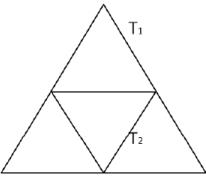Area of triangle T1 =√3 / 4 * (24)2 = 144√3 sq.cm

Area of triangle T2 = 144√3 / 4= 36√3 sq. cm

Sum of the area of all triangles = T1 + T2 + T3 + ...

=>T1 + T1 /4 + T1 /42 + ...

=> T1 / 1 – 0.25

=>4 / 3 * T1

=>4 / 3 * 144√3

=>192 √3

Hence, option D is the correct answer.

QUESTION: 12

If log12 81 = p, then 4 – p / 3(4 + p) is equal to

Solution: Given that: log12 81 = p

=>log81 12 = 1 / p

=> 4 log3 3 ∗ 4 = 1 / p

=> 1 + log34 = 4 / p

Using Componendo and Dividendo,

=>1 + log3 4 – 1 / 1 + log3 4 + 1 = 4 – p / 4 + p

=> log3 4 / 2 + log3 4 = 4 – p / 4 + p

=>log34 / log39 + log34 = 4 – p / 4 + p

=> log34 / log336 = 4 – p / 4 + p

=> 4 – p / 3 * 4 + p = 3 log34 / log336

=>4 – p / 3 * 4 + p = log3 64 / log3 36

=>4 – p / 3 * 4 + p = log36 64

=>4 – p / 3 * 4 + p = log6282 = log68.

Hence, option D is the correct answer.

QUESTION: 13

John borrowed Rs. 2,10,000 from a bank at an interest rate of 10% per annum, compounded annually. The loan was repaid in two equal instalments, the first after one year and the second after another year. The first instalment was interest of one year plus part of the principal amount, while the second was the rest of the principal amount plus due interest thereon. Then each instalment, in Rs., is

Solution: We have to equate the installments and the amount due either at the time of borrowing or at the time when the entire loan is repaid. Let us bring all values to the time frame in which all the dues get settled, i.e., by the end of 2 years.

John borrowed Rs. 2, 10,000 from the bank at 10% per annum. This loan will amount to 2, 10,000*1.1*1.1 = Rs.2, 54,100 by the end of 2 years.

Let the amount paid as installment every year be Rs.x.

John would pay the first installment by the end of the first year. Therefore, we have to calculate the interest on this amount from the end of the first year to the end of the second year. The loan will get settled the moment the second installment is paid.

=> 1.1x + x = 2,54,100

2.1x = 2,54,100

=> x = Rs. 1,21,000.

Therefore, 121000 is the correct answer.

QUESTION: 14

When they work alone, B needs 25% more time to finish a job than A does. They two finish the job in 13 days in the following manner: A works alone till half the job is done, then A and B work together for four days, and finally B works alone to complete the remaining 5% of the job. In how many days can B alone finish the entire job?

Solution: Let us assume that A can complete 'a' units of work in a day and B can complete 'b' units of work in a day.

A works alone till half the work is completed.

A and B work together for 4 days and B works alone to complete the last 5% of the work.

=> A and B in 4 days can complete 45% of the work.

Let us assume the total amount of work to be done to be 100 units.

4a + 4b = 45 --------- (1)

B needs 25% more time than A to finish a job.

=> 1.25*b = a ---------- (2)

Substituting (2) in (1), we get,

5b+4b = 45

9b = 45

b = 5 units/day

B alone can finish the job in 100/5 = 20 days.

Therefore, option A is the right answer.

QUESTION: 15

How many numbers with two or more digits can be formed with the digits 1,2,3,4,5,6,7,8,9, so that in every such number, each digit is used at most once and the digits appear in the ascending order?

Solution: It has been given that the digits in the number should appear in the ascending order. Therefore, there is only 1 possible arrangement of the digits once they are selected to form a number. There are 9 numbers (1,2,3,4,5,6,7,8,9) in total.

2-digit numbers can be formed in 9C2 ways.

3-digit numbers can be formed in 9C3 ways

..................................................

9-digit number can be formed in 9C9 ways.

We know that nC0 + nC1 + nC2 + …………. nCn= 2n

=> 9C0 + 9C1 + 9C2 + ....9C9 = 29

9C0 + 9C1 + ...9C9 = 512

=> We have to subtract 9C0 and 9C1 from both the sides of the equations since we cannot form single digit numbers.

=> 9C2 + 9C3 + ... + 9C9 = 512 − 1 – 9

9C2 + 9C3 + ... + 9C9 = 502

=> Therefore, 502 is the right answer.

QUESTION: 16

A right circular cone, of height 12 ft, stands on its base which has diameter 8 ft. The tip of the cone is cut off with a plane which is parallel to the base and 9 ft from the base. With = 22/7, the volume, in cubic ft, of the remaining part of the cone is

Solution: We are given that diameter of base = 8 ft. Therefore, the radius of circular base = 8/2 = 4 ft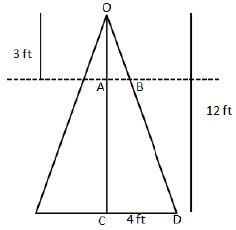In triangle OAB and OCD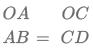=>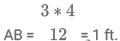Therefore, the volume of remaining part = Volume of entire cone - Volume of smaller cone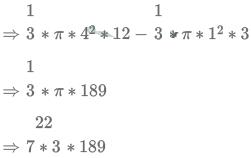=> 198 cubic ft

QUESTION: 17

Raju and Lalitha originally had marbles in the ratio 4:9. Then Lalitha gave some of her marbles to Raju. As a result, the ratio of the number of marbles with Raju to that with Lalitha became 5:6. What fraction of her original number of marbles was given by Lalitha to Raju?

Solution:

Let the number of marbles with Raju and Lalitha initially be 4x and 9x.

Let the number of marbles that Lalitha gave to Raju be a.

It has been given that (4x+a)/ (9x-a) = 5/6

24x + 6a = 45x - 5a

11a = 21x

a/x = 21/11

Fraction of original marbles given to Raju by Lalitha = a/9x (Since Lalitha had 9x marbles initially).

a/9x = 21/99

= 7/33.

Therefore, option D is the right answer.

QUESTION: 18

Each of 74 students in a class studies at least one of the three subjects H, E and P. Ten students study all three subjects, while twenty study H and E, but not P. Every student who studies P also studies H or E or both. If the number of students studying H equals that studying E, then the number of students studying H is

Solution: Let us draw a Venn diagram using the information present in the question.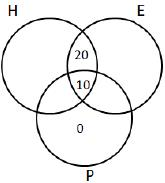It is given that the number of students studying H equals that studying E.

Let 'x' be the total number of students who studied H, and H and P but mot E. We can also say that the same will be the number of students who studied E, and E and P but not H. Therefore,

x + 20 + 10 + x = 74

x = 22

Hence, the number of students studying H = 22 + 10+ 20 = 52

QUESTION: 19

A wholesaler bought walnuts and peanuts, the price of walnut per kg being thrice that of peanut per kg. He then sold 8 kg of peanuts at a profit of 10% and 16 kg of walnuts at a profit of 20% to a shopkeeper. However, the shopkeeper lost 5 kg of walnuts and 3 kg of peanuts in transit. He then mixed the remaining nuts and sold the mixture at Rs. 166 per kg, thus making an overall profit of 25%. At what price, in Rs. per kg, did the wholesaler buy the walnuts?

Solution:

Let the price of peanuts be Rs. 100x per kg

Then, the price of walnuts = Rs. 300x per kg

Cost price of peanuts for the shopkeeper = Rs. 110x per kg

Cost price of walnuts for the shopkeeper = Rs. 360x per kg

Total cost incurred to the shopkeeper while buying = Rs.(8 * 110x + 16 * 360x) = Rs. 6640x

Since, 5kg walnut and 3kg peanuts are lost in transit, the shopkeeper will be remained with (16-5)+(8-3)=16kgs of nuts

Total selling price that the shopkeeper got = Rs. (166 * 16) = Rs. 2656

Profit = 25%

So, cost price = Rs. 2124.80

Therefore, 6640x = 2124.80

On solving, we get x = 0.32

Therefore, price of walnuts = Rs. (300 * 0.32) = Rs. 96 per kg.

Hence, option A is the correct answer

QUESTION: 20

If among 200 students, 105 like pizza and 134 like burger, then the number of students who like only burger can possibly be

Solution:

It has been given that among 200 students, 105 like pizza and 134 like burger.

The question asks us to find out the number of students who can like only burgers among the given values.

The least number of students who like only burger will be obtained when everyone who likes pizza likes burger too.

In this case, 105 students will like pizza and burger and 134-105 = 29 students will like only burger. Therefore, the number of students who like only burger cannot be less than 29.

The maximum number of students who like only burger will be obtained when we try to separate the 2 sets as much as possible.

There are 200 students in total. 105 of them like pizza. Therefore, the remaining 95 students can like only burger and 134-95 = 39 students can like both pizza and burger. As we can see, the number of students who like burger cannot exceed 95.

The number of students who like only burger should lie between 29 and 95 (both the values are included).

93 is the only value among the given options that satisfies this condition and hence, option D is the right answer.

QUESTION: 21

If f(x+2) = f(x) + f(x+1) for all positive integers x, and f(11) = 91, f(15) = 617,then f(10) equals

Solution: f (x + 2) = f (x) + f (x + 1)

As we can see, the value of a term is the sum of the 2 terms preceding it.

It has been given that f(11) = 91 and f(15) = 617.

We have to find the value of f(10).

Let f (10) = b

f (12) = b + 91

f (13) = 91 + b + 91 = 182 + b

f (14) = 182+b+91+b = 273+2b

f (15) = 273+2b+182+b = 455+3b

It has been given that 455+3b = 617

3b = 162

=> b = 54

Therefore, 54 is the correct answer.

QUESTION: 22

In an apartment complex, the number of people aged 51 years and above is 30 and there are at most 39 people whose ages are below 51 years. The average age of all the people in the apartment complex is 38 years. What is the largest possible average age, in years, of the people whose ages are below 51 years?

Solution:

The possible average age of people whose ages are below 51 years will be maximum if the average age of the number of people aged 51 years and above is minimum. Hence, we can say that that there are 30 people having same age 51 years.

Let 'x' be the maximum average age of people whose ages are below 51.

Then we can say that,

51 * 30 + 39 *x

30 39 = 38

⇒ 1530 + 39x = 2622

⇒ x = 1092/39 = 28

Hence, we can say that option D is the correct answer.

QUESTION: 23

A trader sells 10 litres of a mixture of paints A and B, where the amount of B in the mixture does not exceed that of A. The cost of paint A per litre is Rs. 8 more than that of paint B. If the trader sells the entire mixture for Rs. 264 and makes a profit of 10%, then the highest possible cost of paint B, in Rs. per litre, is

Solution:

Let the price of paint B be x.

Price of paint A = x+8

We know that the amount of paint B in the mixture does not exceed the amount of paint A. Therefore, paint B can at the maximum compose 50% of the mixture.

The seller sells 10 litres of paint at Rs.264 earning a profit of 10%.

=> The cost price of 10 litres of the paint mixture = Rs. 240

Therefore, the cost of 1 litre of the mixture = Rs.24

We have to find the highest possible cost of paint B.

When we increase the cost of paint B, the cost of paint A will increase too. If the cost price of the mixture is closer to the cost of paint B,

then the amount of paint B present in the mixture should be greater than the amount of paint A present in the mixture.

The highest possible cost of paint B will be obtained when the volumes of paint A and paint B in the mixture are equal.

=> (x+x+8)/2 = 24

2x = 40

x = Rs. 20

Therefore, option C is the right answer.

QUESTION: 24

In a parallelogram ABCD of area 72 sq cm, the sides CD and AD have lengths 9 cm and 16 cm, respectively. Let P be a point on CD such that AP is perpendicular to CD. Then the area, in sq cm, of triangle APD is

Solution:

In a parallelogram ABCD of area 72 sq cm, the sides CD and AD have lengths 9 cm and 16 cm, respectively. Let P be a point on CD such that AP is perpendicular to CD. Then the area, in sq cm, of triangle APD is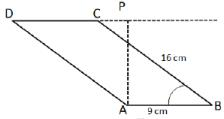Given that area of parallelogram = 72 sq cm

Area of triangle ABC = ½ *area of parallelogram

(1/2)*AB*BC*sinABC = ½ *72

sinABC = ½

∠ABC = 30°

Let us draw a perpendicular CQ from C to AB.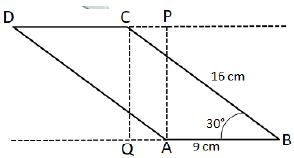∠ B = ∠ D = 30 (opposite angles in a parallelogram)

∠ QCB = ∠ DAP = 60( Sum of angles in a triangle = 180)

In triangles APD and CQB, AP= CQ , AD=CB ( opposite sides of a parallelogram) ,

∠ QCB = ∠ DAP = 60

Hence APD and CQB are congruent triangles using SAS property.

Therefore, we can say that area of triangle APD = area of triangle CQB

In right angle triangle CQB,

QB = CBcos30° = 16 * √3/2 = 8√3cm

CQ = CBsin30° = 16 * 1/2 = 8cm

Therefore, area of triangle CQB = 1/2*CQ*QB = ½ * 8 * 8√3 = 32√3

Hence, we can say that area of triangle APD = 32√3.

QUESTION: 25

If U2 + (U – 2V - 1)2 = −4V (U+V), then what is the value of U+ 3V?

Solution:

Given that U2 + (U − 2V − 1)2 = - 4V (U + V)

⇒U2 + (U − 2V − 1)(U − 2V − 1) = -4V (U + V )

⇒U2 + (U2 − 2UV − U − 2UV + 4V2 + 2V − U + 2V + 1) = - 4V (U + V )

⇒U2 + (U2 − 4UV − 2U + 4V2 + 4V + 1) = -4V (U + V )

⇒ 2U2 − 4UV − 2U + 4V2 + 4V + 1 = −4UV − 4V2

⇒2U2 − 2U + 8V2 + 4V + 1 = 0

⇒ 2[U2 − U + 1/4 ] + 8[V2 + V/2 + 1/6 ] = 0

⇒ 2(U – 1/2)2 + 8(V + 1/4 )2 = 0

Sum of two square terms is zero i.e. individual square term is equal to zero.

U – ½ =0 and V + ¼ = 0

U = ½ and V = 1/-4

Therefore, U + 3V = ½ + (-1*3)/4 = -1/4.

Hence, option C is the correct answer.

QUESTION: 26

In a circle with center O and radius 1 cm, an arc AB makes an angle 60 degrees at O. Let R be the region bounded by the radii OA, OB and the arc AB. If C and D are two points on OA and OB, respectively, such that OC = OD and the area of triangle OCD is half that of R, then the length of OC, in cm, is

Solution:

It is given that radius of the circle = 1 cm

Chord AB subtends an angle of 60° on the centre of the given circle. R be the region bounded by the radii OA, OB and the arc AB.

Therefore, R = 60◦/360◦ *Area of the circle = 1/6*π*(1)2 = π/6 sq. cm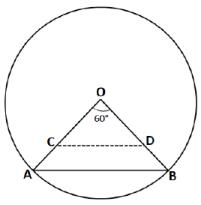It is given that OC = OD and area of triangle OCD is half that of R. Let OC = OD = x.

Area of triangle COD = ½ * OC * OD * sin60◦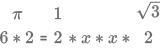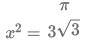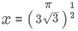cm

Hence, option C is the correct answer.

QUESTION: 27

The distance from A to B is 60 km. Partha and Narayan start from A at the same time and move towards B. Partha takes four hours more than Narayan to reach B. Moreover, Partha reaches the mid-point of A and B two hours before Narayan reaches B. The speed of Partha, in km per hour, is

Solution:

Let the time taken by Partha to cover 60 km be x hours.

Narayan will cover 60 km in x-4 hours.

Speed of Partha = 60/x

Speed of Narayan = 60/(x-4)

Partha reaches the mid-point of A and B two hours before Narayan reaches B.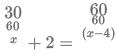⇒ x/2 + 2 = x – 4

⇒ x+4/2 = x-4

x + 4 = 2x − 8 x = 12

Partha will take 12 hours to cross 60 km.

⇒ Speed of Partha = 60/12 = 5 Kmph.

Therefore, option D is the right answer.

QUESTION: 28

Let x, y, z be three positive real numbers in a geometric progression such that x < y < z. If 5x, 16y, and 12z are in an arithmetic progression then the common ratio of the geometric progression is

Solution:

Let x = a, y = ar and z = ar2

It is given that, 5x, 16y and 12z are in AP.

so, 5x + 12z = 32y

On replacing the values of x, y and z, we get

5a + 12ar2 = 32ar

or, 12r2 – 32r + 5 = 0

On solving, r =5/2 or 1/6

For r = 1/6, x < y < z is not satisfied.

So, r=5/2

Hence, option C is the correct answer.

QUESTION: 29

Given that x2018y2017 = 1/2, and x2016y2019= 8, then value of x2 + y3 is

Solution:

Given that x2018y2017 = 1/2 ... (1)

x2016y2019= 8 ... (2)

Equation (2)/ Equation (1)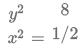y/x = 4 or -4

Case 1: When y/x = 4

x2018(4x)2017 = ½

x2018+2017 (2)2017 = ½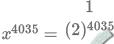x = ½

Since, y/x = 4 ⇒ y=2

Therefore, x2 + y3 = ¼ + 8 = 33/4

Case 2: When y/x = -4

x2018(-4x)2017 = ½

x2018+2017 (2)4034 = -1/2

x4035 = (1/-2)4035x = -1/2

Since, , => x/y = -4 ⇒ y=2

Therefore, x2 + y3 = ¼ + 8 = 33/4 .

Hence, option D is the correct answer.

QUESTION: 30

A tank is fitted with pipes, some filling it and the rest draining it. All filling pipes fill at the same rate, and all draining pipes drain at the same rate. The empty tank gets completely filled in 6 hours when 6 filling and 5 draining pipes are on, but this time becomes 60 hours when 5 filling and 6 draining pipes are on. In how many hours will the empty tank get completely filled when one draining and two filling pipes are on?

Solution: Let the efficiency of filling pipes be 'x' and the efficiency of draining pipes be '-y'.

In the first case,

Capacity of tank = (6x - 5y) * 6..........(i)

In the second case,

Capacity of tank = (5x - 6y) * 60.....(ii)

On equating (i) and (ii), we get

(6x - 5y) * 6 = (5x - 6y) * 60

or, 6x - 5y = 50x - 60y

or, 44x = 55y

or, 4x = 5y

or, x = 1.25y

Capacity of the tank = (6x - 5y) * 6 = (7.5y - 5y) * 6 = 15y

Net efficiency of 2 filling and 1 draining pipes = (2x - y) = (2.5y - y) = 1.5y

Time required = 15y / 1.5y hours = 10 hours.

Hence, 10 is the correct answer.

QUESTION: 31

If log2 (5 + log3 a) = 3 and log5 (4a + 12 + log2 b) = 3, then a + b is equal to

Solution:

log2(5 + log3a) = 3

=>5 + log3 a – 8

=> log3 a = 3

or a = 27

log5 (4a + 12 + log2 b) = 3

=>4a + 12 + log2b = 125

Putting a = 27, we get

log2 b = 5

or, b = 32

So, a + b = 27 + 32 = 59

Hence, option A is the correct answer.

QUESTION: 32

A CAT aspirant appears for a certain number of tests. His average score increases by 1 if the first 10 tests are not considered, and decreases by 1 if the last 10 tests are not considered. If his average scores for the first 10 and the last 10 tests are 20 and 30, respectively, then the total number of tests taken by him is

Solution: Let the total number of tests be 'n' and the average by 'A'

Total score = n*A

When 1st 10 tests are excluded, decrease in total value of scores = (nA - 20 * 10) = (nA - 200)

Also, (n - 10)(A + 1) = (nA - 200)

On solving, we get 10A - n = 190..........(i)

When last 10 tests are excluded, decrease in total value of scores = (nA - 30 * 10) = (nA - 300)

Also, (n - 10)(A - 1) = (nA - 300)

On solving, we get 10A + n = 310..........(ii)

From (i) and (ii), we get n = 60

Hence, 60 is the correct answer.

QUESTION: 33

The number of integers x such that 0.25 < 2X< 200 and 2X + 2 is perfectly divisible by either 3 or 4, is

Solution: At x = 0, 2X = 1 which is in the given range [0.25,200]

2X + 2 = 1 + 2 = 3 Which is divisible by 3. Hence, x = 0 is one possible solution.

At x = 1, 2X = 2 which is in the given range [0.25, 200]

2x + 2 = 2 + 2 = 3 Which is divisible by 4. Hence, x = 1 is one possible solution.

At x = 2, 2X = 4 which is in the given range [0.25, 200]

2x + 2 = 4 + 2 = 6 which is divisible by 3. Hence, x = 2 is one possible solution.

At x = 3, 2X = 8 which is in the given range [0.25, 200]

2x + 2 = 8 + 2 = 3 which is not divisible by 3 or 4. Hence, x = 3 can't be a solution.

At x = 4, 2X = 16 which is in the given range [0.25,200]

2X + 2 = 16 + 2 = 18 Which is divisible by 3. Hence, x = 4 is one possible solution.

At x = 5, 2X = 32 which is in the given range [0.25,200]

2X + 2 = 32 + 2 = 34 Which is not divisible by 3 or 4. Hence, x = 5 can't be a solution.

At x = 6, 2X = 64 which is in the given range [0.25,200]

2X + 2 = 64 + 2 = 66 Which is divisible by 3. Hence, x = 6 is one possible solution.

At x = 7, 2X = 128 which is in the given range [0.25,200]

2X + 2 = 128 + 2 = 130 Which is not divisible by 3 or 4. Hence, x = 7 can't be a solution.

At x = 8, 2X = 256 which is not in the given range [0.25, 200]. Hence, x can't take any value greater than 7.

Therefore, all possible values of x = {0,1,2,4,6}. Hence, we can say that 'x' can take 5 different integer values.

QUESTION: 34

In an examination, the maximum possible score is N while the pass mark is 45% of N. A candidate obtains 36 marks, but falls short of the pass mark by 68%. Which one of the following is then correct?

Solution:

Total marks = N

Pass marks = 45% of N = 0.45N

Marks obtained = 36

It is given that, obtained marks is 68% less than that pass marks

=>the obtained marks is 32% of the pass marks.

So, 0.32 * 0.45N = 36

On solving, we get N = 250

Hence, option B is the correct answer.